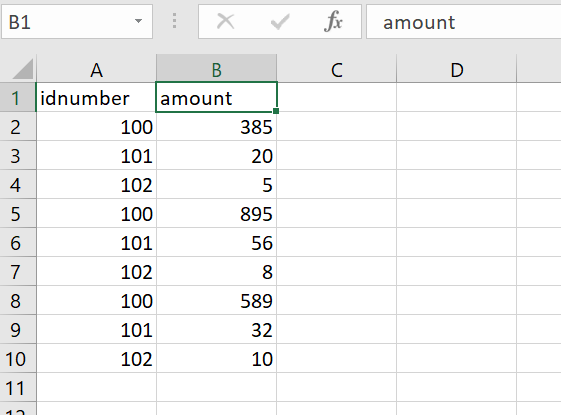# Group data and sum only one of the groups in excel

Good afternoon friends;

One query please, I would like to just add the amounts as long as the “Idnumber” is 100 and store that result in a variable.

For the example I have I should only consider the following:

100 385
100,895
100 589

And the result would be 1869I have tried to adapt this procedure of @ppr , but I can’t understand it very well

Thank you very much in advance

filtro.zip (80.0 KB)

Hi @Lynx ,

Could you also show us what is the Output you are receiving currently and How do you want the Output to be ? Do you only want the Sum value corresponding to Id Number 100 or do you need it in a row/Datatable format ?

If you need only the Sum, then you could try the below :

``````DT.AsEnumerable.Where(Function(x)x("idnumber").ToString.Equals("100")).Sum(Function(x)CDbl(x("amount").ToString))
``````

Let us know if this doesn’t work or is not the Expected outcome.

1 Like

Hi @Lynx

The group by you have used will get all the unique item of id’s and the sum of the amount column based on the I’d

If you want to add only value 100 you can check with @supermanPunch suggestion

And also you try the following
After using the group by you have used
You can use lookup datatable activity to search for value 100 and get target column cell value 1869

Hope this helps

Regards
Sudharsan

I agree to Arpan
when we group with above LINQ we get in the result all distinct Idnumbers with its sums

When for any reason the grouping /summing up is to do along with a filter restriction to only 100 we can do:

strFilter = “100”

dtResult =

``````(From d in dtData.AsEnumerable
Group d by k=d("idnumber").toString.Trim into grp=Group
Where k.Equals(strFilter)
Let s = grp.Sum(Function (x) Convert.ToDouble(x("amount").toString.Trim))
Let ra = new Object(){k, s}
``````

But here we should handle the empty filter result also as described here:

As an alternate we can also create a dictionary for all idnumbers and sums as by following:

dictLK | Dictionary (Of String, Double) =

``````(From d in dtData.AsEnumerable
Group d by k=d("idnumber").toString.Trim into grp=Group
Let s = grp.Sum(Function (x) Convert.ToDouble(x("amount").toString.Trim))
Let ra = new Object(){k, s}
Select  t  = Tuple.Create(k,s)).ToDictionary(Function (t) t.Item1,Function (t) t.Item2)
``````
2 Likes

Yes, my output is just the sum, this data has to be stored in a variable. I don’t want to add anything in the excel.

my output should be 1869, that data, then I will enter it into a mail template.

filtro.zip (87.8 KB)

1 Like

Hi @Lynx
Try this:

``````Datatable1.AsEnumerable.Where(Function(x) x("col1").ToString.Equals("100")).Sum(Function(y) Cint(y("col2")))
``````

Just change col1 and col2 with your column names.

Let me know if that works for you1 Like

Perfect, thank you very much @Emira and also thank you all for your answers, they have helped me a lot.

1 Like

This topic was automatically closed 3 days after the last reply. New replies are no longer allowed.